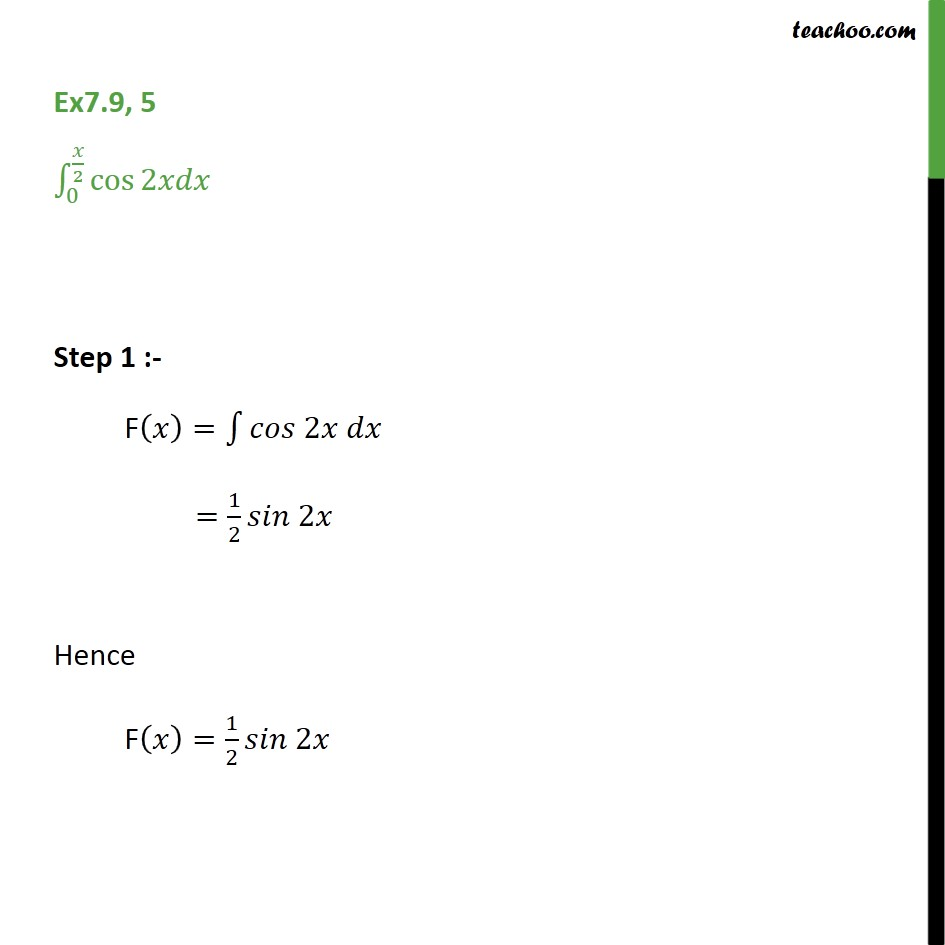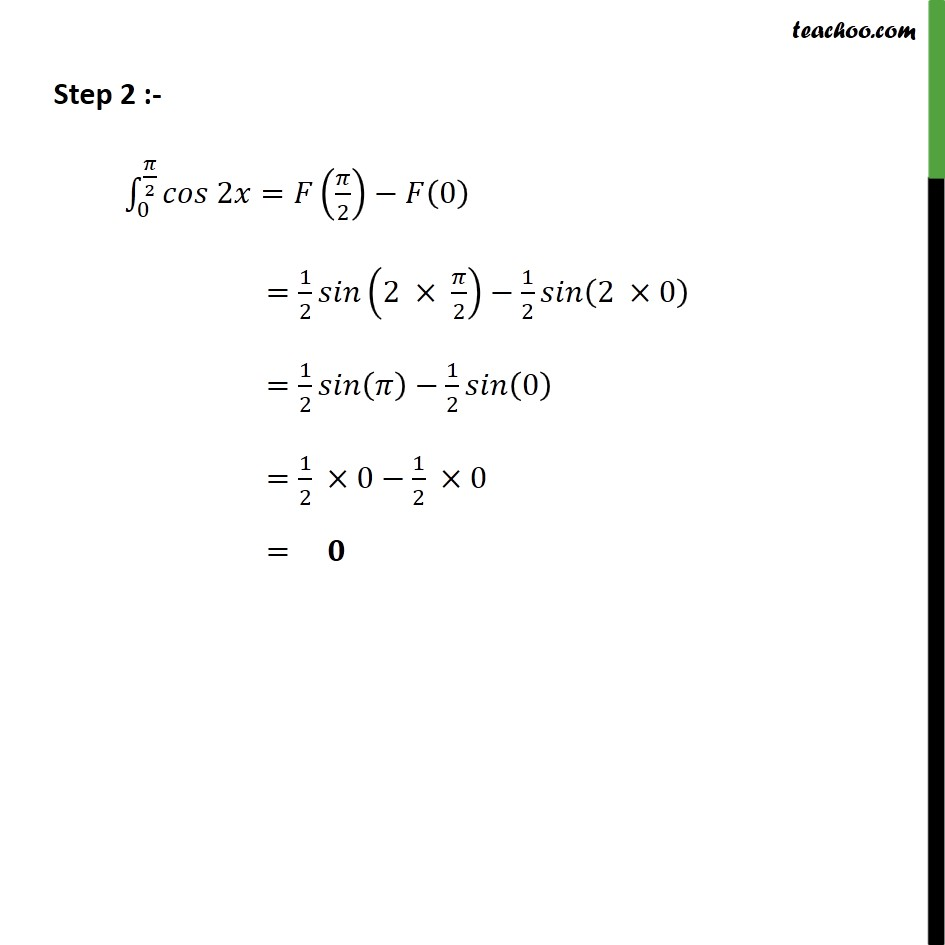1. Chapter 7 Class 12 Integrals
2. Concept wise
3. Definite Integration - By Formulae

Transcript

Ex7.9, 5 0 2 cos 2 Step 1 :- F = 2 = 1 2 2 Hence F = 1 2 2 Step 2 :- 0 2 2 = 2 0 = 1 2 2 2 1 2 2 0 = 1 2 1 2 0 = 1 2 0 1 2 0 =

Definite Integration - By Formulae